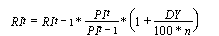# question

Accepted
16 1 1 3

## Returning only certain mnemonics in a different currency

My code:

```mnemonic = ["TTOCOMP","S&PCOMP","MSEAFEL", "MSEMKFL"]
data_type = ["PI","RI"]
start_date = "2019-04-17"
end_date = "TODAY"
frequency = "D"

df = ds.get_data(tickers=mnemonic,
fields=data_type,
start=start_date,
end=end_date,
freq=frequency)
```

How should I modify the above to have the "RI" data return in Canadian dollars?

Thank youUp to 2 attachments (including images) can be used with a maximum of 512.0 KiB each and 1.0 MiB total.

Accepted
681 2 2 1

A return index is available for a range of sector and market indices, including Datastream Global Indices. The return index represents the theoretical aggregate growth in value of the constituents of the index. The index constituents are deemed to return an aggregate daily dividend which is included as an incremental amount to the daily change in price index.

The calculation is as follows:Where:= return index on day t= return index on previous day= price index on day t= price index on previous day

DY = dividend yield of the price index

n = number of days in financial year (normally 260)

Currency conversion can be used with ~Up to 2 attachments (including images) can be used with a maximum of 512.0 KiB each and 1.0 MiB total.

Thank you for the quick reply! One last thing, would you know why the dataframe being created in the above code has each column name as a set? For example, the first column name is ('TTOCOMP(PI)', '').

Instead, I would like the name to be 'TTOCOMP(PI)'

thank you!

Never mind, I figured out a solution. DSWS returns a pandas dataframe object with a multilevel column index. The first level is composed of my mnemonics, while the second level remains empty. I use the following to change this multilevel object to the usual dataframe I want to work with:

`df.columns = df.columns.get_level_values(0)`